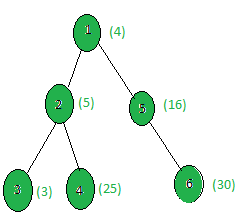# Count the nodes whose weight is a perfect square

• Last Updated : 20 Apr, 2021

Given a tree, and the weights of all the nodes, the task is to count the number of nodes whose weight is a perfect Square.
Examples:

Input:Output:
Only the weights of nodes 1, 4 and 5 are perfect squares.

Approach: Perform dfs on the tree and for every node, check if it’s weight is a perfect square or not.
Below is the implementation of the above approach:

## C++

 `// C++ implementation of the approach` `#include ` `using` `namespace` `std;`   `int` `ans = 0;`   `vector<``int``> graph;` `vector<``int``> weight(100);`   `// Function that returns true` `// if n is a perfect square` `bool` `isPerfectSquare(``int` `n)` `{` `    ``double` `x = ``sqrt``(n);` `    ``if` `(``floor``(x) != ``ceil``(x))` `        ``return` `false``;` `    ``return` `true``;` `}`   `// Function to perform dfs` `void` `dfs(``int` `node, ``int` `parent)` `{` `    ``// If weight of the current node` `    ``// is a perfect square` `    ``if` `(isPerfectSquare(weight[node]))` `        ``ans += 1;`   `    ``for` `(``int` `to : graph[node]) {` `        ``if` `(to == parent)` `            ``continue``;` `        ``dfs(to, node);` `    ``}` `}`   `// Driver code` `int` `main()` `{` `    ``int` `x = 15;`   `    ``// Weights of the node` `    ``weight = 4;` `    ``weight = 5;` `    ``weight = 3;` `    ``weight = 25;` `    ``weight = 16;` `    ``weight = 30;`   `    ``// Edges of the tree` `    ``graph.push_back(2);` `    ``graph.push_back(3);` `    ``graph.push_back(4);` `    ``graph.push_back(5);` `    ``graph.push_back(6);`   `    ``dfs(1, 1);`   `    ``cout << ans;`   `    ``return` `0;` `}`

## Java

 `// Java implementation of the approach` `import` `java.util.*;`   `class` `GFG{` ` `  `static` `int` `ans = ``0``;` ` `  `static` `Vector[] graph = ``new` `Vector[``100``]; ` `static` `int``[] weight = ``new` `int``[``100``];` ` `  `// Function that returns true` `// if n is a perfect square` `static` `boolean` `isPerfectSquare(``int` `n)` `{` `    ``double` `x = Math.sqrt(n);` `    ``if` `(Math.floor(x) != Math.ceil(x))` `        ``return` `false``;` `    ``return` `true``;` `}` ` `  `// Function to perform dfs` `static` `void` `dfs(``int` `node, ``int` `parent)` `{` `    ``// If weight of the current node` `    ``// is a perfect square` `    ``if` `(isPerfectSquare(weight[node]))` `        ``ans += ``1``;` ` `  `    ``for` `(``int` `to : graph[node]) {` `        ``if` `(to == parent)` `            ``continue``;` `        ``dfs(to, node);` `    ``}` `}` ` `  `// Driver code` `public` `static` `void` `main(String[] args)` `{` `    ``int` `x = ``15``;` `    ``for` `(``int` `i = ``0``; i < ``100``; i++) ` `        ``graph[i] = ``new` `Vector<>();` `    `  `    ``// Weights of the node` `    ``weight[``1``] = ``4``;` `    ``weight[``2``] = ``5``;` `    ``weight[``3``] = ``3``;` `    ``weight[``4``] = ``25``;` `    ``weight[``5``] = ``16``;` `    ``weight[``6``] = ``30``;` ` `  `    ``// Edges of the tree` `    ``graph[``1``].add(``2``);` `    ``graph[``2``].add(``3``);` `    ``graph[``2``].add(``4``);` `    ``graph[``1``].add(``5``);` `    ``graph[``5``].add(``6``);` ` `  `    ``dfs(``1``, ``1``);` ` `  `    ``System.out.print(ans); ` `}` `}`   `// This code is contributed by Rajput-Ji`

## Python3

 `# Python3 implementation of the approach` `from` `math ``import` `*` `ans ``=` `0`   `graph ``=` `[[] ``for` `i ``in` `range``(``100``)]` `weight ``=` `[``0``] ``*` `100`   `# Function that returns true` `# if n is a perfect square` `def` `isPerfectSquare(n):` `    ``x ``=` `sqrt(n)` `    ``if` `(floor(x) !``=` `ceil(x)):` `        ``return` `False` `    ``return` `True`   `# Function to perform dfs` `def` `dfs(node, parent):` `    ``global` `ans` `    `  `    ``# If weight of the current node ` `    ``# is a perfect square` `    ``if` `(isPerfectSquare(weight[node])):` `        ``ans ``+``=` `1``;` `    `  `    ``for` `to ``in` `graph[node]:` `        ``if` `(to ``=``=` `parent):` `            ``continue` `        ``dfs(to, node)`   `# Driver code`   `x ``=` `15`   `# Weights of the node` `weight[``1``] ``=` `4` `weight[``2``] ``=` `5` `weight[``3``] ``=` `3` `weight[``4``] ``=` `25` `weight[``5``] ``=` `16` `weight[``6``] ``=` `30`   `# Edges of the tree` `graph[``1``].append(``2``)` `graph[``2``].append(``3``)` `graph[``2``].append(``4``)` `graph[``1``].append(``5``)` `graph[``5``].append(``6``)`   `dfs(``1``, ``1``)` `print``(ans)`   `# This code is contributed by SHUBHAMSINGH10`

## C#

 `// C# program for the above approach` `using` `System; ` `using` `System.Collections; ` `using` `System.Collections.Generic; ` `using` `System.Text; `   `class` `GFG{` `    `  `static` `int` `ans = 0;`   `static` `ArrayList[] graph = ``new` `ArrayList; ` `static` `int``[] weight = ``new` `int``;`   `// Function that returns true` `// if n is a perfect square` `static` `bool` `isPerfectSquare(``int` `n)` `{` `    ``double` `x = Math.Sqrt(n);` `    `  `    ``if` `(Math.Floor(x) != Math.Ceiling(x))` `        ``return` `false``;` `        `  `    ``return` `true``;` `}`   `// Function to perform dfs` `static` `void` `dfs(``int` `node, ``int` `parent)` `{` `    `  `    ``// If weight of the current node` `    ``// is a perfect square` `    ``if` `(isPerfectSquare(weight[node]))` `        ``ans += 1;`   `    ``foreach``(``int` `to ``in` `graph[node]) ` `    ``{` `        ``if` `(to == parent)` `            ``continue``;` `            `  `        ``dfs(to, node);` `    ``}` `}` `    `  `// Driver Code` `public` `static` `void` `Main(``string``[] args)` `{` `    ``//int x = 15;` `    ``for``(``int` `i = 0; i < 100; i++) ` `        ``graph[i] = ``new` `ArrayList();` `    `  `    ``// Weights of the node` `    ``weight = 4;` `    ``weight = 5;` `    ``weight = 3;` `    ``weight = 25;` `    ``weight = 16;` `    ``weight = 30;`   `    ``// Edges of the tree` `    ``graph.Add(2);` `    ``graph.Add(3);` `    ``graph.Add(4);` `    ``graph.Add(5);` `    ``graph.Add(6);`   `    ``dfs(1, 1);`   `    ``Console.Write(ans); ` `}` `}`   `// This code is contributed by rutvik_56`

## Javascript

 ``

Output:

`3`

Complexity Analysis:

• Time Complexity: O(N*logV) where V is the maximum weight of a node in the tree.
In DFS, every node of the tree is processed once and hence the complexity due to the DFS is O(N) for N nodes in the tree. Also, while processing every node, in order to check if the node value is a perfect square or not, the inbuilt sqrt(V), is being called where V is the weight of the node and this function has a complexity of O(log V). Hence for every node, there is an added complexity of O(log V). Therefore, the total time complexity is O(N*logV).
• Auxiliary Space: O(1).
Any extra space is not required, so the space complexity is constant.

My Personal Notes arrow_drop_up
Recommended Articles
Page :